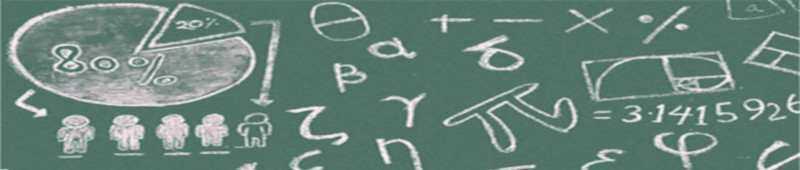The courses taught at the Department of Mathematics, by a physical presence in greek/english (if it's necessary) language, fall into the following categories:

• Compulsory (C): Students must take all these courses (English being one of them) during their undergraduate studies.
• Elective-compulsory (E/C): These courses are characterized as elective-compulsory in the sense that a student is obliged to select at least 7 of them so as to accumulate the 28 credits required.
• Optional (O):Students can choose to attend as many of these courses as they wish in order to accumulate the rest of the credits they need for the successful completion of their four-year degree.

COURSES PER SEMESTER

SEMESTER A

 Course Category Lecture hours Tutoring hours Lab hours ECTS Calculus I [-C-] 4 2 9 Set and Numbers [-C-] 4 2 9 Fundamentals of Mathematics [-C-] 4 2 9 Elementary Analytic Geometry [-O-] 3 4.5

SEMESTER B

 Course Category Lecture hours Tutoring hours Lab hours ECTS Linear Algebra I [-C-] 4 2 9 Introduction to Computer Science [-C-] 4 2 9 Calculus II [-C-] 4 2 9 English I [-C-] 1 2 3

SEMESTER C

 Course Category Lecture hours Tutoring hours Lab hours ECTS Linear Algebra II [-C-] 4 2 9 Topology of Metric Spaces [-C-] 4 2 9 Calculus III [-C-] 4 2 9 English II [-C-] 1 2 3 Programming Languages [-E/C-] 3 1 6 Discrete Mathematics [-E/C-] 4 6 Geometry of Space [-E/C-] 4 6

SEMESTER D

 Course Category Lecture hours Tutoring hours Lab hours ECTS Algebra [-C-] 4 2 9 Ordinary Differential Equations I [-C-] 4 2 9 Calculus IV [-C-] 4 2 9 English IIΙ [-C-] 1 2 3 Linear Programming [-E/C-] 3 1 6 Axiomatic Geometry [-E/C-] 4 6

SEMESTER E

 Course Category Lecture hours Tutoring hours Lab hours ECTS Numerical Analysis [-C-] 3 1 2 9 Analysis [-C-] 4 2 9 Physics I [-C-] 4 2 9 Didactics of Mathematics [-E/C-] 4 6 Number Theory [-E/C-] 4 6 History of Euclidean and non-Euclidean geometries [-E/C-] 4 6 Ordinary Differential Equations II [-O-] 3 4.5 Mathematical Software [-O-] 2 1 4.5 Elementary Set Theory [-O-] 3 4.5 Hyperbolic Geometry [-O-] 3 4.5

SEMESTER F

 Course Category Lecture hours Tutoring hours Lab hours ECTS Probabilities Ι [-C-] 4 2 9 Complex Analysis [-C-] 4 2 9 Differential Geometry [-C-] 4 2 9 Classical Mechanics [-E/C-] 4 6 Mathematical Logic [-E/C-] 4 6 Group Theory [-E/C-] 4 6 Galois Theory [-E/C-] 4 6 Mathematical Modelling [-E/C-] 4 6 Cryptography [-E/C-] 4 6 Didactics of Euclidean Geometry [-E/C-] 4 6 Algebraic Topology [-O-] 3 4.5 Mathematics for the Secondary Education [-O-] 3 4.5

SEMESTER G

 Course Category Lecture hours Tutoring hours Lab hours ECTS Partial Differential Equations [-E/C-] 4 6 Statistics [-E/C-] 4 6 History of Mathematics [-E/C-] 4 6 Scientific Calculation [-E/C-] 3 1 6 New Technologies in Education [-E/C-] 2 2 6 Field Practice (Teaching)Field Practice (Organizations) [-E/C-] 4 6 Fourier Analysis [-E/C-] 4 6 Probability II [-E/C-] 4 6 Microeconomics I [-E/C-] 3 6 Dynamic Programming [-O-] 3 4.5 Dissertation or Undergraduate Thesis [-O-] 13.5 Special Topics of Mathematics I [-O-] 3 4.5 Differentiable Manifolds [-O-] 3 4.5 Groups and Metric Spaces [-O-] 3 4.5 Theory and Practice of Teaching [-O-] 3 4.5

SEMESTER Η

 Course Category Lecture hours Tutoring hours Lab hours ECTS General Topology [-E/C-] 4 6 Functional Analysis [-E/C-] 4 6 Stochastic Processes [-E/C-] 4 6 Databases I [-E/C-] 3 2 6 Physics II [-E/C-] 4 6 Convex Geometry [-E/C-] 4 6 Advanced Programming Languages [-E/C-] 2 2 6 Field Practice (Teaching)Field Practice (Organizations) [-E/C-] 4 6 Macroeconomic Theory I [-E/C-] 3 6 Dissertation or Undergraduate Thesis [-O-] 13.5 Topics in Geometry [-O-] 3 4.5 Topics in Analysis [-O-] 3 4.5 Coding Theory [-O-] 3 4.5 History for Mathematics Education [-O-] 3 4.5 Topics in Algebra [-O-] 3 6 Special Topics of Mathematics II [-O-] 3 4.5 Asymptotic Analysis [-O-] 3 4.5 Theory and Practice of Teaching [-O-] 3 4.5